## Friday, 8 August 2014

### CHAPTER 21 -Properties of Cross Product

We now note some important properties of the cross product:
(i) If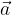$\vec a$ and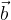$\vec b$ are parallel, their cross product is zero, i.e.$\vec a \times \vec b = \vec 0$
since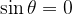$\sin {\rm{\theta }} = 0$. Conversely, if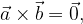$\vec a \times \vec b = \vec 0,$ then$\vec a$ and$\vec b$ must be parallel.
(ii) The cross product is not commutative. In fact,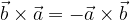$\vec b \times \vec a = - \vec a \times \vec b$
This is because the direction of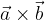$\vec a \times \vec b$ was defined so that$\vec a$$\vec b$ and$\vec a \times \vec b$ form a right handed system(iii) The cross product is distributive over vector addition: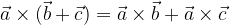$\vec a \times (\vec b + \vec c) = \vec a \times \vec b + \vec a \times \vec c$ and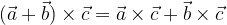$(\vec a + \vec b) \times \vec c = \vec a \times \vec c + \vec b \times \vec c$
(iv)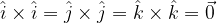$\hat i \times \hat i = \hat j \times \hat j = \hat k \times \hat k = \vec 0$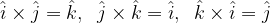$\hat i \times \hat j = \hat k,\;\;\hat j \times \hat k = \hat i,\;\;\hat k \times \hat i = \hat j$
These relations can be remembered asGoing in the reverse direction, we have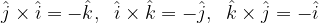$\hat j \times \hat i = - \hat k,\;\;\hat i \times \hat k = - \hat j,\;\;\hat k \times \hat j = - \hat i$
Thus, for two vectors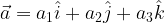$\vec a = {a_1}\hat i + {a_2}\hat j + {a_3}\hat k$ and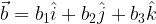$\vec b = {b_1}\hat i + {b_2}\hat j + {b_3}\hat k$ we have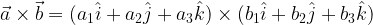$\vec a \times \vec b = ({a_1}\hat i + {a_2}\hat j + {a_3}\hat k) \times ({b_1}\hat i + {b_2}\hat j + {b_3}\hat k)$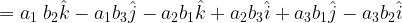$= {a_1}\;{b_2}\hat k - {a_1}{b_3}\hat j - {a_2}{b_1}\hat k + {a_2}{b_3}\hat i + {a_3}{b_1}\hat j - {a_3}{b_2}\hat i$$= \hat i({a_2}{b_3} - {a_3}{b_2}) + \hat j({a_3}{b_1} - {a_1}{b_3}) + \hat k({a_1}{b_2} - {a_2}{b_1})$
This can be written concisely in determinant notation as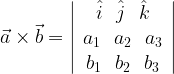$\vec a \times \vec b = \left| {\begin{array}{*{20}{c}} {\hat i}\,\,\,\,{\hat j}\,\,\,\,{\hat k}\\ {{a_1}}\,\,\,\,{{a_2}}\,\,\,\,{{a_3}}\\ {{b_1}}\,\,\,\,{{b_2}}\,\,\,\,{{b_3}} \end{array}} \right|$
(v) The unit vector(s)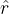$\hat r$ normal to the plane of$\vec a$ and$\vec b$ can be written as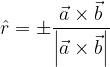$\hat r = \pm \dfrac{{\vec a \times \vec b}}{{\left| {\vec a \times \vec b} \right|}}$